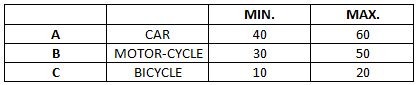### CAT 1997 Question Paper Question 33

Instructions

A certain race is made up of three stretches: A, B and C, each 2 km long, and to be covered by a certain mode of transport. The following table gives these modes of transport for the stretches, and the minimum and maximum possible speeds (in km/hr) over these stretches. The speed over a particular stretch is assumed to be constant. The previous record for the race is 10 min.Question 33

# Mr Tortoise completes the race at an average speed of 20 km/hr. His average speed for the first two stretches is four times that for the last stretch. Find the speed over stretch C.

Solution

Average speed to cover complete race = 20 km/hr = $$\frac{2+2+2}{t_1 + t_2 + t_3}$$ (Where $$t_1$$ is time taken to cover the distance A, $$t_2$$ is time taken to cover the distance B and $$t_3$$ is time taken to cover the distance C)

So total time =$$t_1 + t_2 + t_3 = 18$$ min.

Avg. speed for first two streches = $$\frac{4}{t_1 + t_2}$$

Avg. speed for last strech = $$\frac{2}{t_3}$$

Given: $$\frac{4}{t_1 + t_2} = 4 \times \frac{2}{t_3}$$

Or $$2t_1 + 2t_2 = t_3$$

Or $$t_3 = 12$$ min.

So $$V_3 = \frac{2}{12} \times 60$$ = 10 km/hr

• All Quant CAT Formulas and shortcuts PDF
• 30+ CAT previous papers with solutions PDF

##### Praveen Vm

3 years, 5 months ago

Let y be the distance of the stretch.
For all 3 laps it's the same distance y.
Now , average speed 20 =(y+y+y / t1+t2+t3)
t1,t2,t3 are the time taken in each lap.
Let x be the speed for the last stretch.
1st stretch y=4x(t1)
2nd y=4x(t2)
3rd y=xt3
Now find t1,t2 and t3 from the above eqns. And substitute it inbthe first one.

We get x=10.

##### Nithin Thattil

3 years, 11 months ago

This answer is difficult to understand

##### Praveen Vm

3 years, 5 months ago

🤣Yeah bro

OR